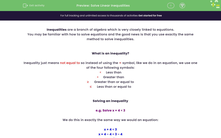# Solve Linear Inequalities

In this worksheet, students will solve linear inequalities where variables will end up on both the right- and left-hand sides. If it ends up on the right, students will need to flip the inequality over and swap the sign.Key stage:  KS 4

Year:  GCSE

GCSE Subjects:   Maths

GCSE Boards:   AQA, Eduqas, OCR, Pearson Edexcel,

Curriculum topic:   Algebra

Curriculum subtopic:   Solving Equations and Inequalities Algebraic Inequalities

Difficulty level:#### Worksheet Overview

Inequalities are a branch of algebra which is very closely linked to equations.

You may be familiar with how to solve equations and the good news is that you use exactly the same method to solve inequalities.

What is an inequality?

Inequality just means not equal to so instead of using the = symbol, like we do in an equation, we use one of the four following symbols:

<     Less than

>     Greater than

≥      Greater than or equal to

≤      Less than or equal to

Solving an inequality

e.g. Solve x + 4 < 3

We do this in exactly the same way we would an equation:

x + 4 < 3

x + 4 - 4 < 3 - 4

x < -1

What this means is that if you put any number that is less than -1 (-2, -3, -4, etc.) into the original equation, it will be true.

e.g. Solve x/2 > 7

Again, we do this in exactly the same way we would an equation:

x/2 > 7

x/2 × 2 > 7 × 2

x > 14

The special case

There is one special case that we need to be aware of and on the lookout for.

Let's look at an example to illustrate this point:

5 < x + 3

5 - 3 < x + 3 - 3

2 < x

The issue here is that the x is on the right.

If this was an equation, we would just swap them around but we cannot do this with an inequality.

The inequality currently means '2 is less than x' so if we want to get our x on the left, we would have to say 'x is greater than 2'.

So 2 < x is the same as x > 2.

The rule is: If you want to switch the sides of an inequality over, remember you have to swap the sign.

In this activity, we will solve inequalities where our variable will end up on both the right- and left-hand sides.

If it ends up on the right, we will need to flip our inequality over and swap our sign.

### What is EdPlace?

We're your National Curriculum aligned online education content provider helping each child succeed in English, maths and science from year 1 to GCSE. With an EdPlace account you’ll be able to track and measure progress, helping each child achieve their best. We build confidence and attainment by personalising each child’s learning at a level that suits them.

Get started